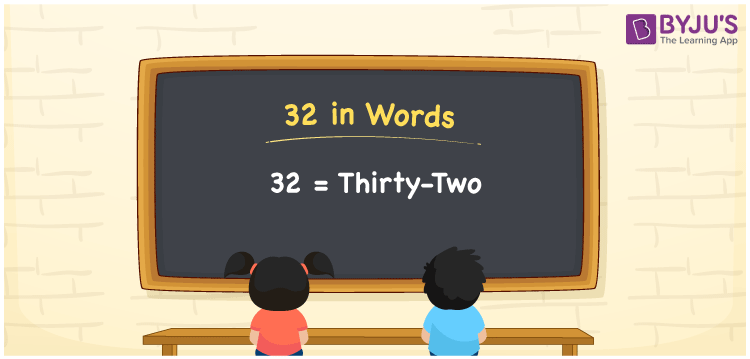# 32 in Words

32 in words is written as “Thirty-two”. In Mathematics, 32 is a natural number that is used to represent a value or a quantity. For example, Mac gave his friend Rs.32 or Mac gave his friend Rupees Thirty-two. Hence, 32 in words is used in different ways in Maths and in real life. Learn more about Numbers In Words at BYJU’S.

 32 in Words Thirty-two Thirty-two in Numerical Form 32

## 32 in English Words

32 in Words using English alphabets is expressed as given below:## How to Write 32 in Words?

We will use here place value method to write 32 in words. The below place value chart for number 32 represents the position of each digit.

 Tens Ones 3 2

From the above table, we can see, that 3 is at tens place, and 2 is at ones place. We need to read the number from right to left in the table. Thus, together we can write the number 32 as Thirty-two.

### Expanded Form of 32

We can write the expanded form of 32 as

3 at Tens + 2 at Ones

= 3 × 10 + 2 × 1

= 32

= Thirty-two

32 is a whole number that is succeeded by 31 and preceded by 33. Find more about the number 32 below:

• 32 in Words – Thirty-two
• Is 32 an odd number? – No
• Is 32 an even number? – Yes
• Is 32 a perfect square number? – No
• Is 32 a perfect cube number? – No
• Is 32 a prime number? – No
• Is 32 a composite number? – Yes

## Frequently Asked Questions on 32 in words

Q1

### What is 32 in words?

32 in words is given by Thirty-two.
Q2

### What is the rule to write 32 in words?

32 in words is written based on the place values of each digit. In 32, 3 is at tens place, and 2 is at units place. Thus, thirty plus two is read as Thirty-two.
Q3

### How to write 32 in English words?

32 in English is written as Thirty-two
Test your Knowledge on 32 in Words